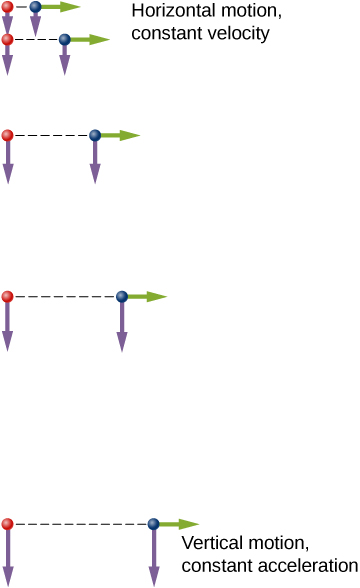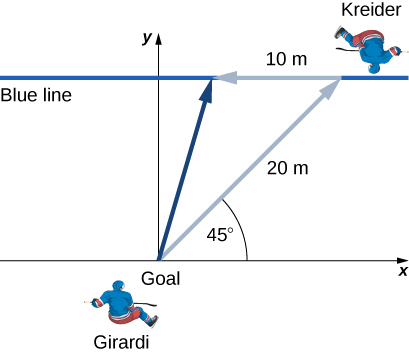4.1 Displacement and velocity vectors  (Page 4/7)

 Page 4 / 7

An example illustrating the independence of vertical and horizontal motions is given by two baseballs. One baseball is dropped from rest. At the same instant, another is thrown horizontally from the same height and it follows a curved path. A stroboscope captures the positions of the balls at fixed time intervals as they fall ( [link] ).A diagram of the motions of two identical balls: one falls from rest and the other has an initial horizontal velocity. Each subsequent position is an equal time interval. Arrows represent the horizontal and vertical velocities at each position. The ball on the right has an initial horizontal velocity whereas the ball on the left has no horizontal velocity. Despite the difference in horizontal velocities, the vertical velocities and positions are identical for both balls, which shows the vertical and horizontal motions are independent.

It is remarkable that for each flash of the strobe, the vertical positions of the two balls are the same. This similarity implies vertical motion is independent of whether the ball is moving horizontally. (Assuming no air resistance, the vertical motion of a falling object is influenced by gravity only, not by any horizontal forces.) Careful examination of the ball thrown horizontally shows it travels the same horizontal distance between flashes. This is because there are no additional forces on the ball in the horizontal direction after it is thrown. This result means horizontal velocity is constant and is affected neither by vertical motion nor by gravity (which is vertical). Note this case is true for ideal conditions only. In the real world, air resistance affects the speed of the balls in both directions.

The two-dimensional curved path of the horizontally thrown ball is composed of two independent one-dimensional motions (horizontal and vertical). The key to analyzing such motion, called projectile motion , is to resolve it into motions along perpendicular directions. Resolving two-dimensional motion into perpendicular components is possible because the components are independent.

Summary

• The position function $\stackrel{\to }{r}\left(t\right)$ gives the position as a function of time of a particle moving in two or three dimensions. Graphically, it is a vector from the origin of a chosen coordinate system to the point where the particle is located at a specific time.
• The displacement vector $\text{Δ}\stackrel{\to }{r}$ gives the shortest distance between any two points on the trajectory of a particle in two or three dimensions.
• Instantaneous velocity gives the speed and direction of a particle at a specific time on its trajectory in two or three dimensions, and is a vector in two and three dimensions.
• The velocity vector is tangent to the trajectory of the particle.
• Displacement $\stackrel{\to }{r}\left(t\right)$ can be written as a vector sum of the one-dimensional displacements $\stackrel{\to }{x}\left(t\right),\stackrel{\to }{y}\left(t\right),\stackrel{\to }{z}\left(t\right)$ along the x , y , and z directions.
• Velocity $\stackrel{\to }{v}\left(t\right)$ can be written as a vector sum of the one-dimensional velocities ${v}_{x}\left(t\right),{v}_{y}\left(t\right),{v}_{z}\left(t\right)$ along the x , y , and z directions.
• Motion in any given direction is independent of motion in a perpendicular direction.

Conceptual questions

What form does the trajectory of a particle have if the distance from any point A to point B is equal to the magnitude of the displacement from A to B ?

straight line

Give an example of a trajectory in two or three dimensions caused by independent perpendicular motions.

If the instantaneous velocity is zero, what can be said about the slope of the position function?

The slope must be zero because the velocity vector is tangent to the graph of the position function.

Problems

The coordinates of a particle in a rectangular coordinate system are (1.0, –4.0, 6.0). What is the position vector of the particle?

$\stackrel{\to }{r}=1.0\stackrel{^}{i}-4.0\stackrel{^}{j}+6.0\stackrel{^}{k}$

The position of a particle changes from ${\stackrel{\to }{r}}_{1}=\left(2.0\text{​}\stackrel{^}{i}+3.0\stackrel{^}{j}\right)\text{cm}$ to ${\stackrel{\to }{r}}_{2}=\left(-4.0\stackrel{^}{i}+3.0\stackrel{^}{j}\right)\phantom{\rule{0.2em}{0ex}}\text{cm}.$ What is the particle’s displacement?

The 18th hole at Pebble Beach Golf Course is a dogleg to the left of length 496.0 m. The fairway off the tee is taken to be the x direction. A golfer hits his tee shot a distance of 300.0 m, corresponding to a displacement $\text{Δ}{\stackrel{\to }{r}}_{1}=300.0\phantom{\rule{0.2em}{0ex}}\text{m}\stackrel{^}{i},$ and hits his second shot 189.0 m with a displacement $\text{Δ}{\stackrel{\to }{r}}_{2}=172.0\phantom{\rule{0.2em}{0ex}}\text{m}\stackrel{^}{i}+80.3\phantom{\rule{0.2em}{0ex}}\text{m}\stackrel{^}{j}.$ What is the final displacement of the golf ball from the tee?

$\text{Δ}{\stackrel{\to }{r}}_{\text{Total}}=472.0\phantom{\rule{0.2em}{0ex}}\text{m}\stackrel{^}{i}+80.3\phantom{\rule{0.2em}{0ex}}\text{m}\stackrel{^}{j}$

A bird flies straight northeast a distance of 95.0 km for 3.0 h. With the x -axis due east and the y -axis due north, what is the displacement in unit vector notation for the bird? What is the average velocity for the trip?

A cyclist rides 5.0 km due east, then 10.0 km $20\text{°}$ west of north. From this point she rides 8.0 km due west. What is the final displacement from where the cyclist started?

$\text{Sum of displacements}=-6.4\phantom{\rule{0.2em}{0ex}}\text{km}\stackrel{^}{i}+9.4\phantom{\rule{0.2em}{0ex}}\text{km}\stackrel{^}{j}$

New York Rangers defenseman Daniel Girardi stands at the goal and passes a hockey puck 20 m and $45\text{°}$ from straight down the ice to left wing Chris Kreider waiting at the blue line. Kreider waits for Girardi to reach the blue line and passes the puck directly across the ice to him 10 m away. What is the final displacement of the puck? See the following figure.The position of a particle is $\stackrel{\to }{r}\left(t\right)=4.0{t}^{2}\stackrel{^}{i}-3.0\stackrel{^}{j}+2.0{t}^{3}\stackrel{^}{k}\text{m}.$ (a) What is the velocity of the particle at 0 s and at $1.0$ s? (b) What is the average velocity between 0 s and $1.0$ s?

a. $\stackrel{\to }{v}\left(t\right)=8.0t\stackrel{^}{i}+6.0{t}^{2}\stackrel{^}{k},\phantom{\rule{0.7em}{0ex}}\stackrel{\to }{v}\left(0\right)=0,\phantom{\rule{0.7em}{0ex}}\stackrel{\to }{v}\left(1.0\right)=8.0\stackrel{^}{i}+6.0\stackrel{^}{k}\text{m/s}$ ,
b. ${\stackrel{\to }{v}}_{\text{avg}}=4.0\text{​}\stackrel{^}{i}+2.0\stackrel{^}{k}\phantom{\rule{0.2em}{0ex}}\text{m/s}$

Clay Matthews, a linebacker for the Green Bay Packers, can reach a speed of 10.0 m/s. At the start of a play, Matthews runs downfield at $45\text{°}$ with respect to the 50-yard line and covers 8.0 m in 1 s. He then runs straight down the field at $90\text{°}$ with respect to the 50-yard line for 12 m, with an elapsed time of 1.2 s. (a) What is Matthews’ final displacement from the start of the play? (b) What is his average velocity?

The F-35B Lighting II is a short-takeoff and vertical landing fighter jet. If it does a vertical takeoff to 20.00-m height above the ground and then follows a flight path angled at $30\text{°}$ with respect to the ground for 20.00 km, what is the final displacement?

$\text{Δ}{\stackrel{\to }{r}}_{1}=20.00\phantom{\rule{0.2em}{0ex}}\text{m}\stackrel{^}{j},\text{Δ}{\stackrel{\to }{r}}_{2}=\left(2.000\phantom{\rule{0.2em}{0ex}}×\phantom{\rule{0.2em}{0ex}}{10}^{4}\text{m}\right)\phantom{\rule{0.2em}{0ex}}\left(\text{cos}30\text{°}\stackrel{^}{i}+\text{sin}\phantom{\rule{0.2em}{0ex}}30\text{°}\stackrel{^}{j}\right)$
$\text{Δ}\stackrel{\to }{r}=1.700\phantom{\rule{0.2em}{0ex}}×\phantom{\rule{0.2em}{0ex}}{10}^{4}\text{m}\stackrel{^}{i}+1.002\phantom{\rule{0.2em}{0ex}}×\phantom{\rule{0.2em}{0ex}}{10}^{4}\text{m}\stackrel{^}{j}$

a particle projected from origin moving on x-y plane passes through P & Q having consituents (9,7) , (18,4) respectively.find eq. of trajectry.
definition of inertia
the reluctance of a body to start moving when it is at rest and to stop moving when it is in motion
charles
An inherent property by virtue of which the body remains in its pure state or initial state
Kushal
why current is not a vector quantity , whereas it have magnitude as well as direction.
why
daniel
the flow of current is not current
fitzgerald
bcoz it doesn't satisfy the algabric laws of vectors
Shiekh
The Electric current can be defined as the dot product of the current density and the differential cross-sectional area vector : ... So the electric current is a scalar quantity . Scalars are related to tensors by the fact that a scalar is a tensor of order or rank zero .
Kushal
what is binomial theorem
what is binary operations
Tollum
What is the formula to calculat parallel forces that acts in opposite direction?
position, velocity and acceleration of vector
hi
peter
hi
daniel
hi
Vedisha
*a plane flies with a velocity of 1000km/hr in a direction North60degree east.find it effective velocity in the easterly and northerly direction.*
imam
hello
Lydia
hello Lydia.
Sackson
What is momentum
isijola
hello
A rail way truck of mass 2400kg is hung onto a stationary trunk on a level track and collides with it at 4.7m|s. After collision the two trunk move together with a common speed of 1.2m|s. Calculate the mass of the stationary trunk
I need the solving for this question
philip
is the eye the same like the camera
I can't understand
Suraia
Josh
I think the question is that ,,, the working principal of eye and camera same or not?
Sardar
yes i think is same as the camera
what are the dimensions of surface tension
samsfavor
why is the "_" sign used for a wave to the right instead of to the left?
why classical mechanics is necessary for graduate students?
classical mechanics?
Victor
principle of superposition?
principle of superposition allows us to find the electric field on a charge by finding the x and y components
Kidus
Two Masses,m and 2m,approach each along a path at right angles to each other .After collision,they stick together and move off at 2m/s at angle 37° to the original direction of the mass m. What where the initial speeds of the two particles
MB
2m & m initial velocity 1.8m/s & 4.8m/s respectively,apply conservation of linear momentum in two perpendicular directions.
Shubhrant
A body on circular orbit makes an angular displacement given by teta(t)=2(t)+5(t)+5.if time t is in seconds calculate the angular velocity at t=2s
MB
2+5+0=7sec differentiate above equation w.r.t time, as angular velocity is rate of change of angular displacement.
Shubhrant
Ok i got a question I'm not asking how gravity works. I would like to know why gravity works. like why is gravity the way it is. What is the true nature of gravity?
gravity pulls towards a mass...like every object is pulled towards earth
Ashok
An automobile traveling with an initial velocity of 25m/s is accelerated to 35m/s in 6s,the wheel of the automobile is 80cm in diameter. find * The angular acceleration
(10/6) ÷0.4=4.167 per sec
Shubhrant
what is the formula for pressure?
force/area
Kidus
force is newtom
Kidus
and area is meter squared
Kidus
so in SI units pressure is N/m^2
Kidus
In customary United States units pressure is lb/in^2. pound per square inch
KidusByBy